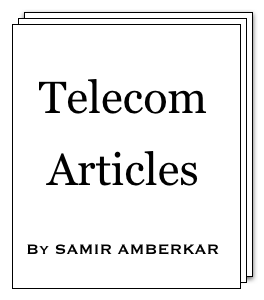online LTE test online C test Updated or New CDF explained5G Page5G Intent (a presentation)5G Intent (article)CV2X PageA Look at CV2X (a presentation)

 About Feedback Information Theory Modulation Multiple Access OSI Model Data Link layer SS7 Word about ATM GSM GPRS UMTS WiMAX LTE 5G Standard Reference Reference books Resources on Web Miscellaneous CV2X Mind Map Perl resources Magic MSC tool Bar graph tool C programming ASCII table Project Management Simple Google box HTML characters Site view counter 7 5 0 , 5 4 0 (May-2010 till Mar-2019)Case of Frequency Correction Burst

Case of Frequency Correction Burst [Under GSM > GMSK modulation]

Here is format of Frequency Correction Burst (3GPP TS 45.002:5.2.4).

 Tail bits 3 Fixed bits 142 Tail bits 3 Guard period 8.25

Tail bits and Fixed bits are all "0". Let us look at how the carrier waveform looks like with the help of equations illustrated in earlier article.

Carrier waveform is represented as:

____
x(t') = (
 2Ec T
) . cos(2πf0t' + φ(t') + φ0)

The phase difference is also function of t and αi (differentially encoded bits):

 φ(t') = n . t'-iT . Σ ( αiπh . ∫ g(u) du ) i=1 . -∞ .

αi = { +1, -1 }
h = 0.5

Let us look at φ(t') with the help of waveforms. We would separate out πh and draw φ(t') for 1 bit, 2 bits, 3 bits, and 4 bits transfer (each bit "0"):If you observe, all waveforms start at value close to (-number of bits/2), becomes almost straight line of slope (1/T) and then saturates on (+number of bits/2). If we approximate φ(t'), we could re-write it as below:

φ(t') = πh . t'/T = 2π.(1/4T).t'

Carrier for frequency correction burst can be written as:

cos(2π(f0 + 1/4T)t')

Thus FCCH would look like non-modulated wave of frequency (1/4T=)66.7 kHz higher than carrier frequency !!!

In next article, we will solve the modulation equations (from earlier article).

References: GSM book by Mouly and Pautet.